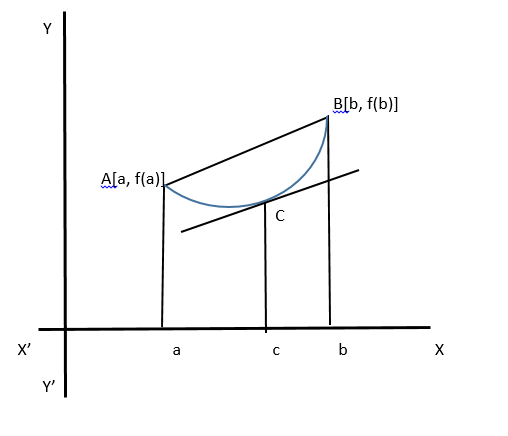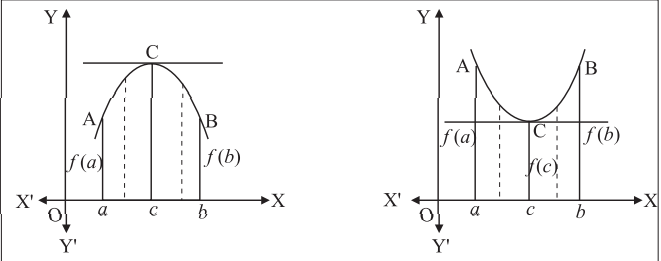GFG App
Open AppBrowser
Continue

# Mean value theorem – Advanced Differentiation | Class 12 Maths

If y = f(x) is a real-valued function defined on [a, b] such that

• f(x) is continuous on [a, b]
• f(x) is differentiable on (a, b), then there exist at least one point c ∈ (a, b) such that f(b) – f(a) = f’(c)(b – a)

It is Also called Lagrange’s mean value theorem or extended mean value theorem.

### Geometrical Significance

We draw the curve y = f(x) and take the points A(a, f(a)) and B(b, f(b)) on the curve, then

Slope of a chord AB = (f(b) – f(a))/(b – a)

Since,

f’(c) = (f(b) – f(a))/(b – a)            (Statement of Mean Value Theorem)

f’(c) = Slope of chord AB

This shows that the tangent to the curve y = f(x) at the point x = c is parallel to the chord AB.## Rolle’s Theorem

If f(a) = f(b) then,

there exists a real number c ∈ (a, b) such that f'(c) = 0, This special case called as Rolle’s theorem.

Rolle’s theorem essentially states that any real-valued differential function that attains equal values at two distinct points on it, must have at least one stationary point somewhere in between them, that is a point where the first derivative (the slope of the tangent line to the graph of a function) is zero.

### Geometrical Significance

Let f(x) is a real-valued function defined on [a, b] and it is continuous on [a, b].  This means that we can draw the graph of f(x) between the value of x = a and x = b also f(x) is differentiable on (a, b) which means the graph of f(x) has a tangent at each point of (a, b). Now the existence of real number c ∈ (a.b) such that f'(c) = 0 shows that the tangent to the curve at x = c has slope 0, that is tangent is parallel to x-axis since f(a) = f(b).### Sample Problems on Rolle’s Theorem

Problem 1: Verify Rolle’s Theorem for the Following Function: f (x) = x2 – 5x + 9 on [1, 4]

Solution:

Since, f(x) is Polynomial in  x

f(x) is continuous on [1. 4]

f(x) is differentiable on (1, 4)

f(1) = 5, f(4) = 42 – 5 * 4 + 9 = 5

Hence, f(0) = f(4) = 5

Thus, all the conditions of Rolle’s Theorem are satisfied.

The derivative of f(x) should vanish for at least one point in c in (0, 4). To obtain the value of c,

We proceed as follows,

f (x) = x2 – 5x + 9

f'(x) =  2x-5⇢

if x =5/2  then f'(x) = 0

Hence, ∃ c = 5/2 in (1, 4)

we know that , 5/2 ∈1, 4)

Thus, Rolle’s Theorem is  verified.

Problem 2: Discuss the applicability of Rolle’s theorem for the function f(x) = x2/3 , x ∈ [-1, 1]

Solution:

f(x) = x2/3 continuous at every  point of [-1, 1]

Now, f(x) = x2/3

f'(x) = 2/3(x-1/3)

As, x ⇢ 0+ f'(x) ⇢ ∞

f'(0) does not exist.

f (x) is not differentiable at  x=0

f (x) is not differentiable in (-1. 1)

Rolle’s theorem is not applicable for the given function.

### Cauchy Mean Value Theorem

Let, f(x) and g(x) are the functions defined on [a, b] such that,

f(x) and g(x) both are continuous on [a, b]

f(x) and g(x) both are differentiable on (a, b), then there exists at least one point c ∈ (a, b) such that

f'(c) {g(b) – g(a)}= g'(c){ f(b) – f(a)}

The case that g(a) = g(b) is easy. So, assume that g(a) ≠ g(b). Define

h(x) = f'(x) {g(b) – g(a)}= g'(x){f(b) – f(a)}

Clearly, h(a) = h(b). Applying Rolle’s Theorem we have that there is a c with a < c < b

Such that h'(x) = f'(c) {g(b) – g(a)}= g'(c){f(b) – f(a)}

For this c we have that,

f'(c) {g(b) – g(a)} = g'(c){f(b) – f(a)}

The classical Mean Value Theorem is a special case of Cauchy’s Mean Value Theorem.

It is the case when g(x) ≡ x. The Cauchy Mean Value Theorem can be used to prove L’Hospital’s Theorem.

### Applications of Mean Value Theorem

• Mean value theorem is the fundamental theorem of calculus.
• Physical interpretation(like speed analysis).
• If the derivative greater than zero then f is strictly Increasing function.
• Taylor Series and number theory.

## Increasing and Decreasing Function

With the help of mean value theorem, we can find

### Increasing Function

The function y = f(x) is said to be an increasing function of x in the interval (a, b):

if f'(x) > 0 for a x ∈ (a, b)

### Sample Problems

Problem 1: Test whether the following function is increasing or not.

f(x) = x3 – 3x2 + 3x – 100, x ∈ R

Solution:

f(x) = x3 – 3x2 + 3x – 100, x ∈ R

f'(x) = 3x2 – 6x + 3

= 3(x – 1)2

Since (x – 1)2 is always positive x ≠1

f'(x) >0, for all x ∈ R.

Hence, f(x) is an increasing function,  for all x ∈ R.

Problem 2: Find the value of x for which the function f(x) = x3+12x2+36x+6,  x is increasing.

Solution:

f(x) = x3 + 12x2 + 36x + 6

f'(x) = 3x2 + 24x + 36 = 3(x + 2)(x + 6)

Now, f'(x) > 0, as f(x) is increasing

3(x + 2)(x + 6) > 0

Case 1: x + 2 < 0 and x + 6 > 0

x > -2 and x > -6

Case 2: x + 2 < 0 and x + 6 > 0

x < -2 and x < -6

f(x) = x3 + 12x2 + 36x + 6 is increasing if and only if x < -6 or x > -2

Hence, x∈ (-∞, -6) or x∈ (-2, ∞)

### Decreasing Function

The function y = f(x) is said to be a decreasing function of x in the interval (a, b)

If f'(x) < 0 for a x ∈ (a, b).

### Sample Problems

Problem 1: Test if the function f (x) = 2 – 3x + 3x2 – x3 is increasing or decreasing for all x ∈ R

Solution:

f(x) = 2 – 3x + 3x2 – x

f'(x) = -3 + 6x – 3x2

= -3(x2 – 2x + 1)

= -3(x – 1)2

Since (x – 1)2 is always positive, x ≠1

f'(x) < 0, for all x ∈ R.

Hence, f(x) is a decreasing function, for all x ∈ R.

Problem 2: Find the value of x for which the function f(x) = 2x3 – 9x2 + 12x + 2, x is decreasing.

Solution:

f(x) = 2x3 – 9x2 + 12x + 2,

f'(x) = 6x2 – 18x + 12

= 6(x – 1)(x – 2)

Now, f'(x) < 0,

As f(x) is decreasing

6(x – 1)(x – 2) < 0

Case 1: (x – 1) < 0 and x – 2 > 0

x < 1 and x > 2 which is absurd

Case 2: (x  – 1) > 0 and x – 2 < 0

x > 1 and x < 2

1 < x < 2

f(x) = x3 + 12x2 + 36x + 6 is decreasing if and only if x ∈ (1, 2).

### Sample Problems on Mean Value Theorem

Problem 1: f(x) = (x – 1)(x – 2)(x – 3), x ∈ [0, 4]. Find c if Mean value theorem can be applied.

Solution:

f(x) = (x – 1)(x – 2)(x – 3),

x ∈ [0, 4]

f(x) = x3 – 6x2 + 11x – 6

As f(x) is Polynomial in x

• f(x) is continuous on [0. 4]
• f(x) is differentiable on (0, 4)

Thus, all the conditions of Mean Value theorem are satisfied.

To verify theorem we have to find c ∈ (0, 4) such that

f’(c) = (f(4) – f(0))/(4 – 0)                                …(1)

Now, f(4) = (4 – 1)(4 – 2)(4 – 3) = 6

f(0) = (0 – 1)(0 – 2)(0 – 3) = – 6 and

f'(x) = 3x2 – 12x + 11

f'(c) = 3c2 – 12c + 11

(f(4) – f(0))/(4 – 0) = 3c2 – 12c + 11         [From 1]

3 = 3c2 – 12c + 11

3c2 – 12c + 8 = 0

c = 2 ± 2/√3

Both values of c lie between 0 and 4.

Problem 2: Verify LaGrange’s mean value theorem for the function f(x) = log(x) on [1, e]

Solution:

f(x) = log(x)

f(x) is continuous on [1, e]

f(x) is differentiable on (1, e), Thus all the conditions of Mean Value theorem are satisfied.

We want to find c ∈ (1, e) such that

f(e) – f(1) = f'(c) {e – 1}           … from (1) [By mean value theorem]

log e -log 1 = f'(c) {e – 1}

1 – 0 = f'(c) {e – 1}

f'(c) = 1/(e – 1)                      …

Now,

f(x) = log(x)

Hence, f'(x) = 1/x

f'(c) = 1/c           …

From  and 

1/(e – 1) = 1/c

Hence,

c = e – 1 which is lies in interval (1, e)

Thus, LaGrange’s mean value theorem verified.

My Personal Notes arrow_drop_up